Prepare for Boolean Operation

1. prepare for boolean

## Calculate In/Out for each link

All intersections are part of the graph now. This has divided a 2D plane into seperate enclosed areas, that are formed by links and nodes. If we can tell for each enclosed area if it is part of groupA and/or groupB, we can also tell if this enclosed area is part of a certain boolean operation. To achieve this we need to find for links that enclose a particular area if the sides of those links are part of groupA or B. Using a scanbeam we can calculate for each link in a beam, if its right or left side was inside the polygon from which the link originated. After those values are known for all the links in the graph, they will be used to calculate for each link if the left and/or right side was part of the groupA and/or groupB area ( See In/Out GroupA and/or GroupB. ). This information will be used to set flags for each link, to tell if the left or right side of the link is part of a boolean opera

#### In/Out GroupA and/or GroupB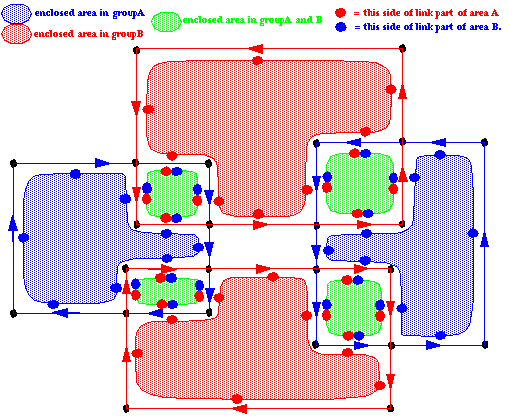## calculate Left Right values

Again we use a scanbeam to calculate the Group Left/Right values for the links in the graph. For each original polygon (links with same graph number) we need to now for both sides of the links if they where inside or outside the original polygon. The method used is based on filling polygons with the "winding method". The principle is to calculate for each enclosed area in a polygon, how many times it is overlapped by the polygon itself ( See self intersecting and overlapping polygon.. ). In red is shown how many times certain areas are overlapping, we could also say: how many times we are in the polygon. The direction of the links can be used to calculate the number of overlaps. The direction of the first link in the beam brings us into the polygon, every next link with the same direction brings us one level deeper into the polygon, the opposite direction one level lower. As can be seen in beam2, ones the number of links to the right and left are eqaul, we are outside the polygon again. No matter what the direction of the following link is, it will bring us into the polygon again.

#### self intersecting and overlapping polygon.Actually the depth itself is not calculated. It is only important to now if the next link will bring us deeper into the polygon or not, the boolean flag "inc" is used for that. Only the new links found at the low node of the current beam need to be calculated. All the graphnumbers of the new links are put in a list, only those graphs/polygons will be recalculated for the current beam to set the inc flag correctly for all links with the same graphnumber.

#### setting inc flagFor a flatbeam this method can not be used, because for all the links at for instance the low node, the Ysp and Ysn will be eqaul. During sorting of the beam records the order of those records in the beam is not fixed. This is why all flatbeams are skipped, still the graphnumber of new links inserted at the low node are recorded. The first coming normal beam will calculate the "inc" again ( See skipping flatbeams.. ). The flatbeam links will be calculated later, after rotating the whole graph 90 degrees in the end, and performing another sweep.

#### skipping flatbeams.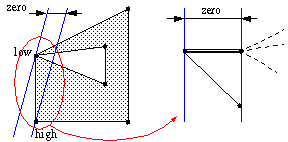## Calculate Group Left Right for each link.

After the calculation of the "inc" flag value of each link, the group left and right values can be calculated.
Each link has four flags: LeftA, RightA, LeftB, RightB

Those flags tell if the Left or Right side of the link is part of groupA or groupB. The principle for calculating those flags, is simular to calculating the "inc" flag for single polygons. Here we need to calculate how many polygons of a certain group are overlapping. If the number of overlaps is more then zero, it is clear that this area is part of the given group. The overlaps are calculated for groupA and groupB. The "inc" flag tells if we go deeper in a polygon of a certain group. If at the same time we where already in another polygon of the same group, we can say that the overlap for this group is "depth" polygon1 + "depth" polygon2. The "inc" + "the group flag" are used to calculate for each side of a link how deep the overlap of an enclosed area on that side of the link is for each group.

The same scanbeam is used for this. Because all intersection in the complete graph are known at this point, we only have to set the group flags once for each link. If the same link is still part of another following beam it does not need to be set again, the values in the next beam should be the same. Simple because the link does not cross any other link, each link is unique. To prevent setting the same flags again we set the mark flag for a link. In case the mark flag is set, we can perform a simplified calculation in the beam for calculating the groupA and groupB depth.

While traversing the scanbeam, the depth for each side of the link for each group is calculated using the "inc" flag of the link. The top side the link will be set based on the current depth of groupA and groupB, the bottom side of the link will be set according to the "inc" flag of the link. The top and bottom side maps to a side of the link depending on its direction (going left or right in the beam). The flags are boolean, if the depth is > zero for a certain group it is set to True else to False.

#### calculate group left right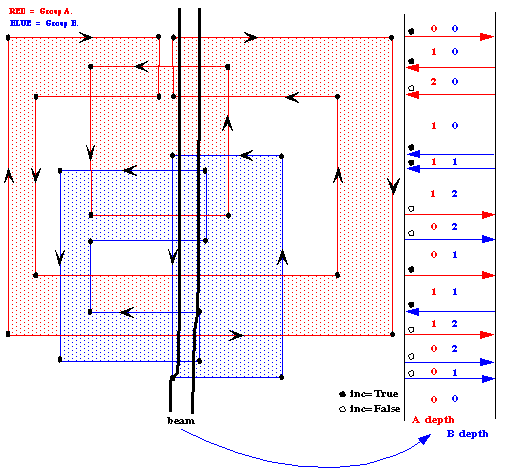## Set operation flags

After all left and right GroupA and GroupB flags are set, we have enough information to tell if a certain link will be part of a boolean operation or not. To make this easy, we first calculate the following help variables:
• BORDER_AB: The link is on the edge of groupA or groupB In a formule based on group flags this is

• (LeftA or LeftB) xor (RightA or RightB)
• BORDER_A: The link is on the edge of GroupA

• (LeftA xor RightA)
• BORDER_B: The link is one the edge of GroupB

• (LeftB xor RightB)
• IN_A: The link is completely inside GroupA

• (LeftA and RightA)
• IN_B: The link is completely inside GroupB

• (LeftB and RightB)
• IN_AB: The link is completely inside GroupA and GroupB

• (LeftA or LeftB) and (RightA or RightB)
Using those variables we can find which links should be part of a certain boolean operation.

### Merge

The merge (GroupB OR GroupB), contains the area that is part of groupA and/or part of groupB. This means take all links that mark the edge of this area .MERGE = BORDER_AB.

### A-Subtract-B

The subtract area for groupA-groupB contains links that or on the border of groupA and not part of groupB, and links that are on the border of groupB and part of groupA..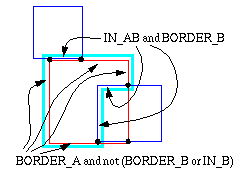A-SUBTRACT-B=(BORDER_A and not(BORDER_B or IN_B) ) or (IN_AB and BORDER_B)

### B-Subtract-A

The subtract area for groupB-groupA contains links that or on the border of groupB and not part of groupA, and links that are on the border of groupA and part of groupB.
B-SUBTRACT-A=(BORDER_B and not(BORDER_A or IN_A) ) or (IN_AB and BORDER_A)

### Intersect

The intersection means (GroupA AND groupB). There are three sets of links to be selected. First the links that have sides in IN_A and also are on the edge of groupB plus the links that are IN_B and at the same time are on the edge of groupA. Next to this there is the case that one group has edges parallel to edges in the other group.This leads to the following formula:(BORDER_B and IN_A) or (BORDER_A and IN_B) or (BORDER_A and BORDER_B and not(IN_AB) )

### Exclusive Or

This the area that is not part of both group at the same time. The result of the previous operations MERGE and INTERSECT can be used to get the right links for this operation..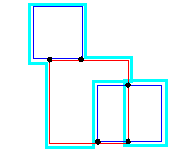(BORDER_AB exor Intersect)

## Remove redundant links

After setting the operation flags, it is possible that some links are not part of any operation, they can be removed from the graph. For the method chosen to extract the result from the graph later on, it is not allowed to have links parallel to eachother. Those sets of parallel links will be reduced to one link for this reason.